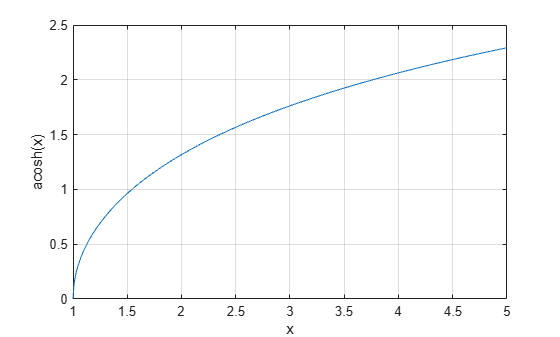# acosh

Inverse hyperbolic cosine

## Syntax

``Y = acosh(X)``

## Description

example

````Y = acosh(X)` returns the inverse hyperbolic cosine of the elements of `X`. The function accepts both real and complex inputs. All angles are in radians.```

## Examples

collapse all

Find the inverse hyperbolic cosine of the elements of vector `X`. The `acosh` function acts on `X` element-wise.

```X = [2 -3 1+2i]; Y = acosh(X)```
```Y = 1×3 complex 1.3170 + 0.0000i 1.7627 + 3.1416i 1.5286 + 1.1437i ```

Plot the inverse hyperbolic cosine function over the interval $1\le x\le 5$.

```x = 1:0.01:5; plot(x,acosh(x)) grid on xlabel('x') ylabel('acosh(x)')```## Input Arguments

collapse all

Hyperbolic cosine of angle, specified as a scalar, vector, matrix, or multidimensional array. The `acosh` operation is element-wise when `X` is nonscalar.

Data Types: `single` | `double`
Complex Number Support: Yes

collapse all

### Inverse Hyperbolic Cosine

For real values $x$ in the domain $x>1$, the inverse hyperbolic cosine satisfies

`${\mathrm{cosh}}^{-1}\left(x\right)=\mathrm{log}\left(x+\sqrt{{x}^{2}-1}\right).$`

For complex numbers $z=x+iy$, as well as real values in the domain $-\text{\hspace{0.17em}}\infty , the call `acosh(z)` returns complex results.